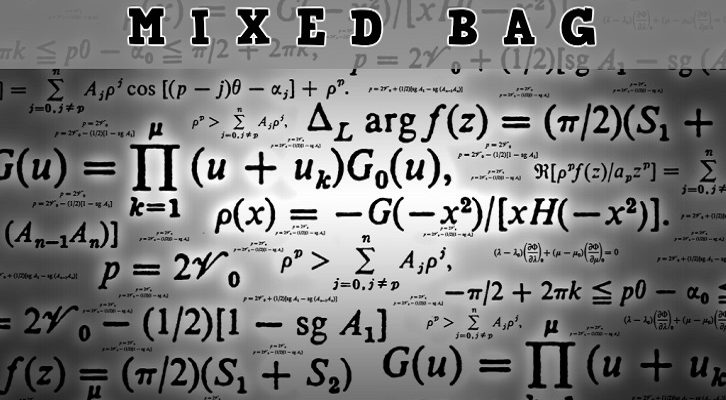# Few more questions – Mixed bag

Have given below four questions across topics. Will discuss the answers shortly.

1. Average of 6 distinct positive integers is 33. The median of the three largest numbers is 43. What is the difference between the highest and lowest possible median of the 6 numbers?

2. In class A, the ratio of boys to girls is 2:3. In class B the ratio of boys to girls is 4 : 5. If the ratio of boys to girls in both classes put together is 3 : 4, what is the ratio of number of girls in class A to number of girls in class B?

3. N is an 80-digit positive integer (in the decimal scale). All digits except the 44th digit (from the left) are 2. If N is divisible by 13, find the 26th digit.

4. A page is torn from a novel. The sum of the remaining digits is 10000. What is the sum of the two page-numbers on the torn page of this novel?